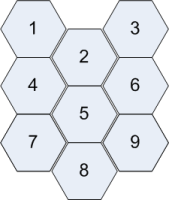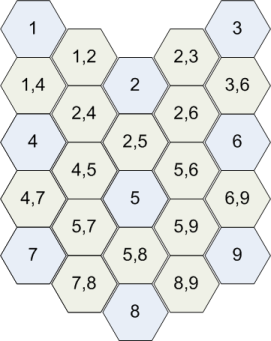2 views

How exactly is a U-matrix constructed in order to visualize a self-organizing-map? More specifically, suppose that I have an output grid of 3x3 nodes (that have already been trained), how do I construct a U-matrix from this? You can e.g. assume that the neurons (and inputs) have dimension 4.

I have found several resources on the web, but they are not clear or they are contradictory. For example, the original paper is full of typos.

by (33.1k points)

A U-matrix is defined as a visual representation of the distances between neurons in the input data dimension space. You can calculate the distance between adjacent neurons, using their trained vector. In your case, the input dimension was 4, then each neuron in the trained map also corresponds to a 4-dimensional vector.

For example:

A 3x3 hexagonal map.You can say that a U-matrix is a 5x5 matrix with interpolated elements for each connection between two neurons similar to the following matrixThe {x,y} elements are the distance between neuron x and y, and the values in {x} elements are the mean of the around values.

For example, {4,5} = distance(4,5) and {4} = mean({1,4}, {2,4}, {4,5}, {4,7}). The calculation of the distance you use the trained 4-dimensional vector of each neuron and the distance (usually Euclidean distance) formula that used can be for the training of the map. So, the values of the U-matrix are only numbers. So, you can assign a light gray color to the largest of these values and a dark gray to the smallest and the other values to corresponding shades of gray.

To gain more insights on U-Matrix. study Machine Learning Course. Also, study Machine Learning Tutorial if you want to master the domain.